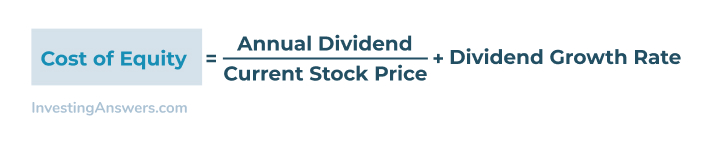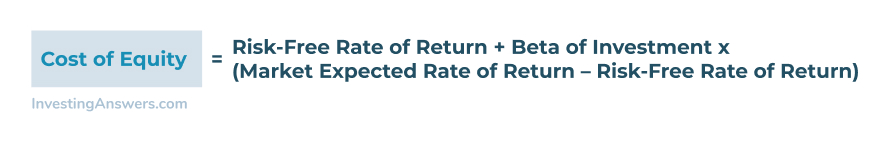# Cost of Equity

Updated July 23, 2021

## What is Cost of Equity?

Cost of equity is the rate of return required on an equity investment by an investor. The cost of equity also refers to the required rate of return on a company's equity investment, such as an acquisition, since it is the return required by the company's investors.

## Cost of Equity Formula

Cost of equity can be calculated two different ways;

The dividend growth model is specific to investments in companies that pay an annual dividend. The CAPM model can be applied to any equity investment, whether or not dividends are paid out.

### Cost of Equity Formula: Dividend Growth Model

The formula for the cost of equity on a dividend-paying equity investment is as follows:This formula is based on the idea that the company's cost of equity is the return it must provide to shareholders. That return can be calculated as a rate of return by taking into account next year's annual dividend amount and dividing it by the current stock price and then adding the expected growth rate of the stock dividend.

### Cost of Equity Formula: Capital Asset Pricing Model (CAPM)

The cost of equity CAPM formula is as follows:This formula takes into account the volatility (Beta) of a company relative to the market and calculates the expected risk when evaluating the cost of equity. It also considers the risk-free rate of return (typically 10-year US treasury notes) when making the calculation.

## Cost of Equity Example

Mark is considering investing in company XYZ and wants to know the cost of equity before investing his money. He calculated the cost of equity using both models to evaluate his potential investment.

### Dividend Growth Model Example

Using the dividend growth model, here's how Mark evaluates XYZs stock:

Cost of Equity = (\$1 dividend / \$20 share price) + 7% expected growth

According to the dividend growth model, the cost of equity when investing in XYZ is 12%.

### Capital Asset Pricing Model (CAPM) Example

Using the dividend growth model, here's how Mark evaluates XYZs stock:

Cost of Equity = 1.5% + 1.1 * (10% - 1.5%)

According to the CAPM, the cost of equity when investing in XYZ is 9.5%.

## Cost of Equity vs Cost of Debt

The cost of debt is typically the interest rate paid for acquiring the debt, which is the lender's expected return, while the cost of equity is based on the shareholder's expected return on investment.

## Cost of Equity vs WACC

A company's capital typically consists of both debt and equity. The weighted average cost of capital (WACC) accounts for the costs of both debt and equity, and the amounts of equity and of debt. It is the "average" return to the company's lenders and shareholders or its "average" cost of capital. The cost of equity is the return only to the company's shareholders and it is the cost of only its equity capital.

## Cost of Equity vs Cost of Capital

The cost of capital includes both equity and debt costs in the evaluation. The cost of capital includes weighing the cost of equity, as well as the cost of debt when looking at a capital purchase (such as acquiring another company).

The cost of debt is typically the interest rate paid on any loans or bonds for the transaction. Overall, the cost of capital can be determined using the Weighted Average Cost of Capital (WACC) formula.

All of our content is verified for accuracy by Rachel Siegel, CFA and our team of certified financial experts. We pride ourselves on quality, research, and transparency, and we value your feedback. Below you'll find answers to some of the most common reader questions about Cost of Equity.
##### Be the first to ask a question

Rachel Siegel, CFA - 141Rachel Siegel, CFACFA Charterholder

Chartered Financial Analyst

Rachel Siegel, CFA is one of the nation's leading experts at ensuring the accuracy of financial and economic text.  Her prestigious background includes over 10 years of experience in creating professional financial certification exams and another 20 years of college-level teaching.